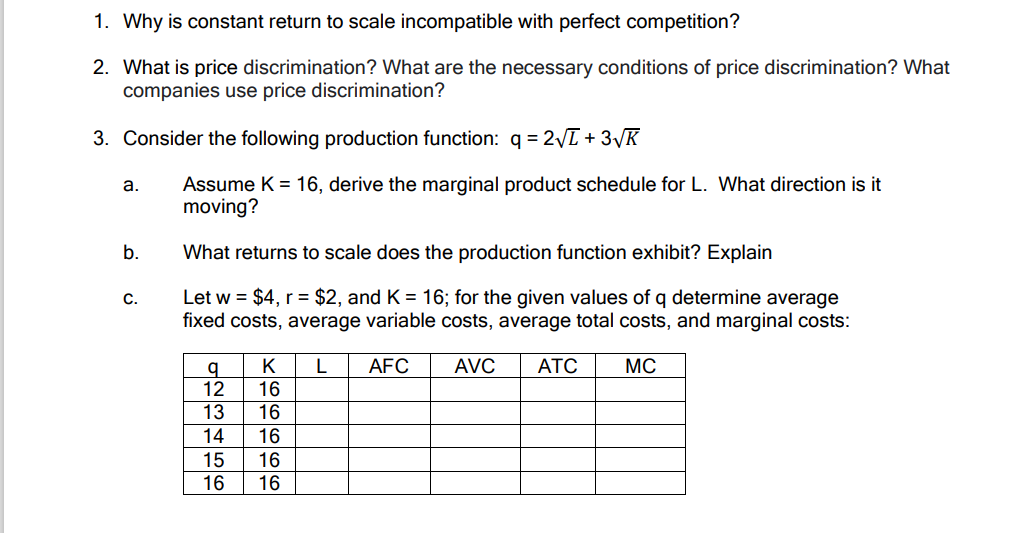### Create an Account

Home / Questions / Why is constant return to scale incompatible with perfect competition? 2. What is price di...

# Why is constant return to scale incompatible with perfect competition? 2. What is price discrimination? What are the necessary conditions of price discrimination? What companies use price discriminati

Why is constant return to scale incompatible with perfect competition? 2. What is price discrimination? What are the necessary conditions of price discrimination? What companies use price discrimination? 3. Consider the following production function: q = 2V1 + 3VK a. Assume K = 16, derive the marginal product schedule for L. What direction is it moving? b. What returns to scale does the production function exhibit? Explain C. Let w = \$4, r = \$2, and K = 16; for the given values of q determine average fixed costs, average variable costs, average total costs, and marginal costs: L AFC AVC ATC MC TIT q 12 13 14 15 16 K 16 16 16 16 16May 01 2021 View more View LessSubscribe To Get Solution